Home > INT3 > Chapter 11 > Lesson 11.2.4 > Problem11-106

11-106.
1. Solve the following problems. Homework Help ✎

1.Write ln(7) = 1.95 in exponential form.

2. Express e5 ≈ 148.41 in logarithmic form.

3. Evaluate ln(e3) without a calculator.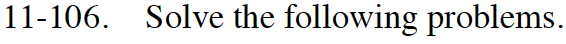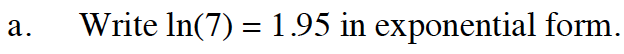e1.95 = 7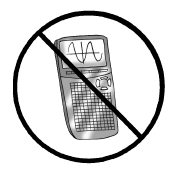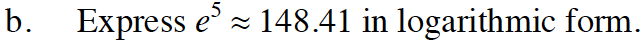Reverse what was done in part (a).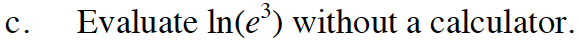The two functions are inverses.